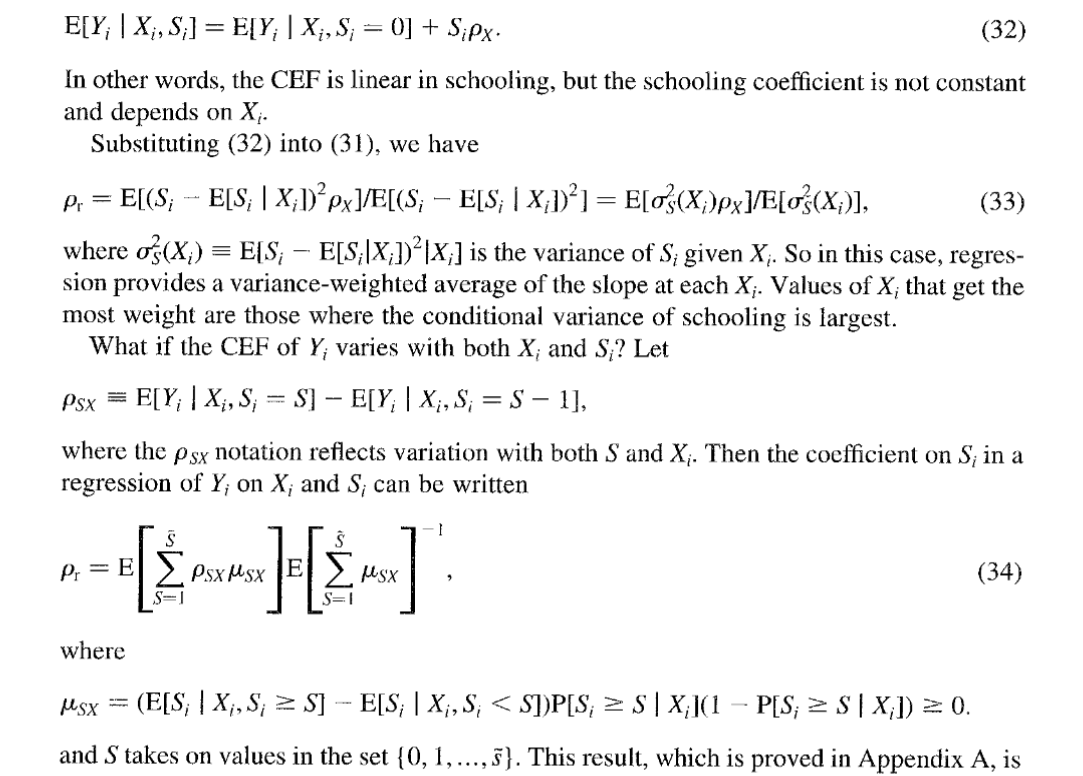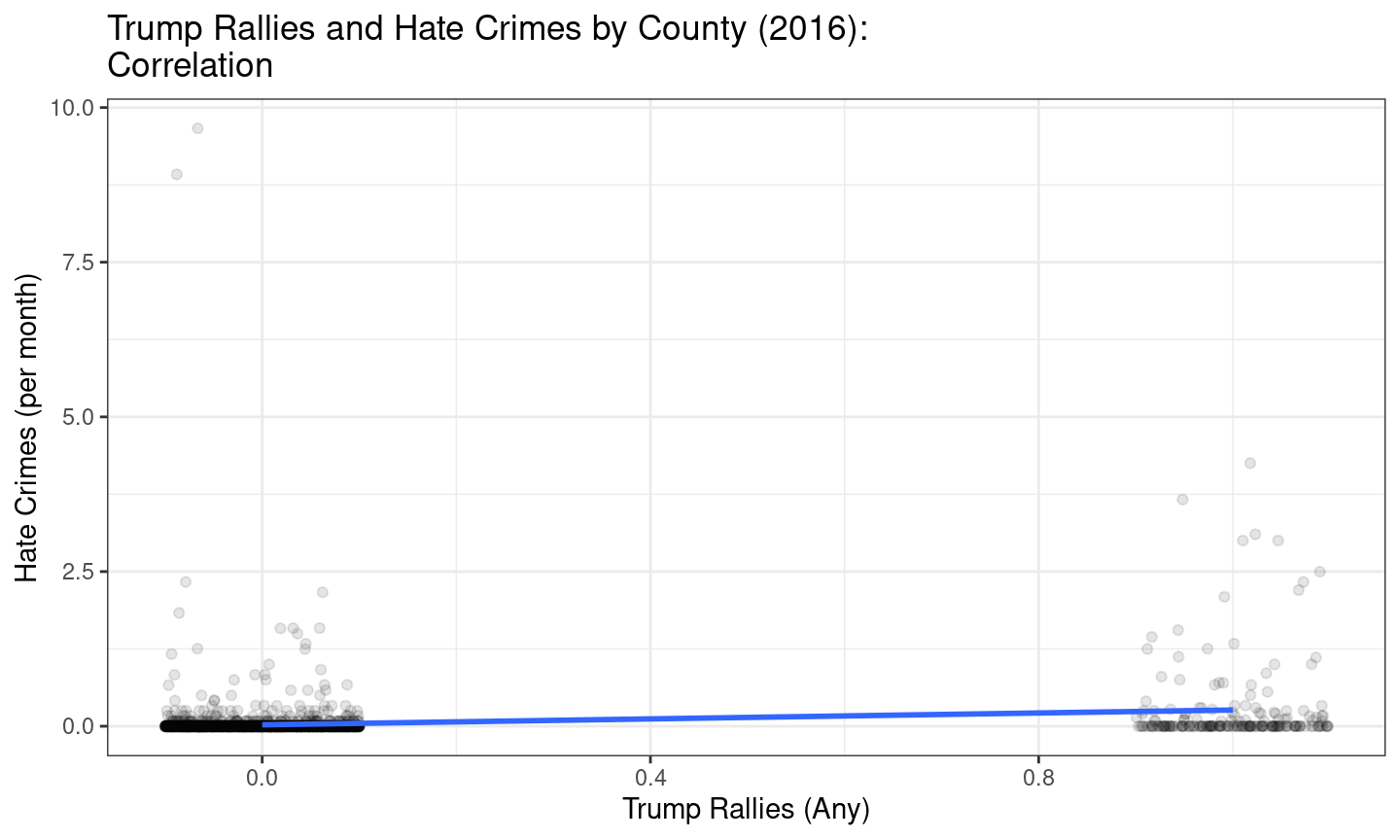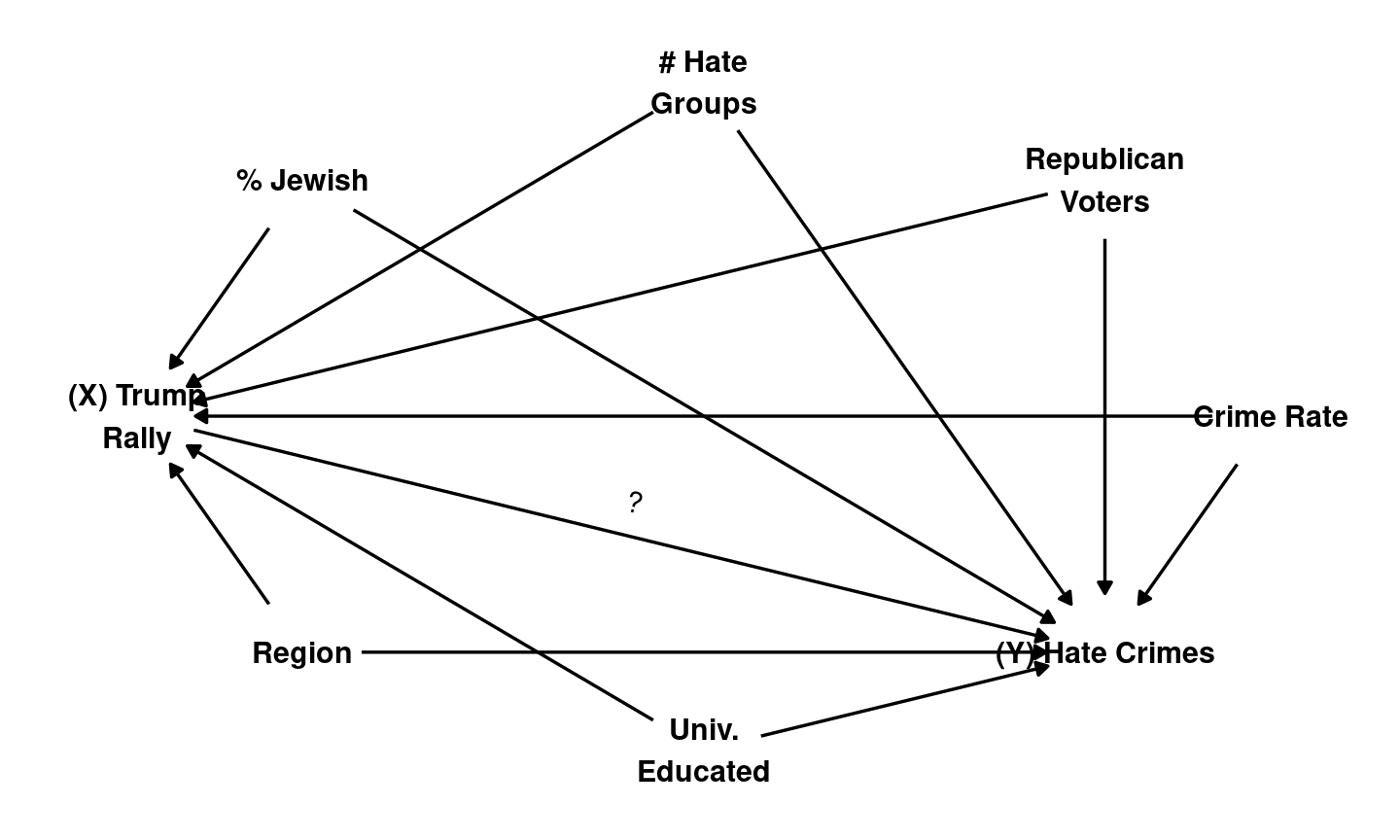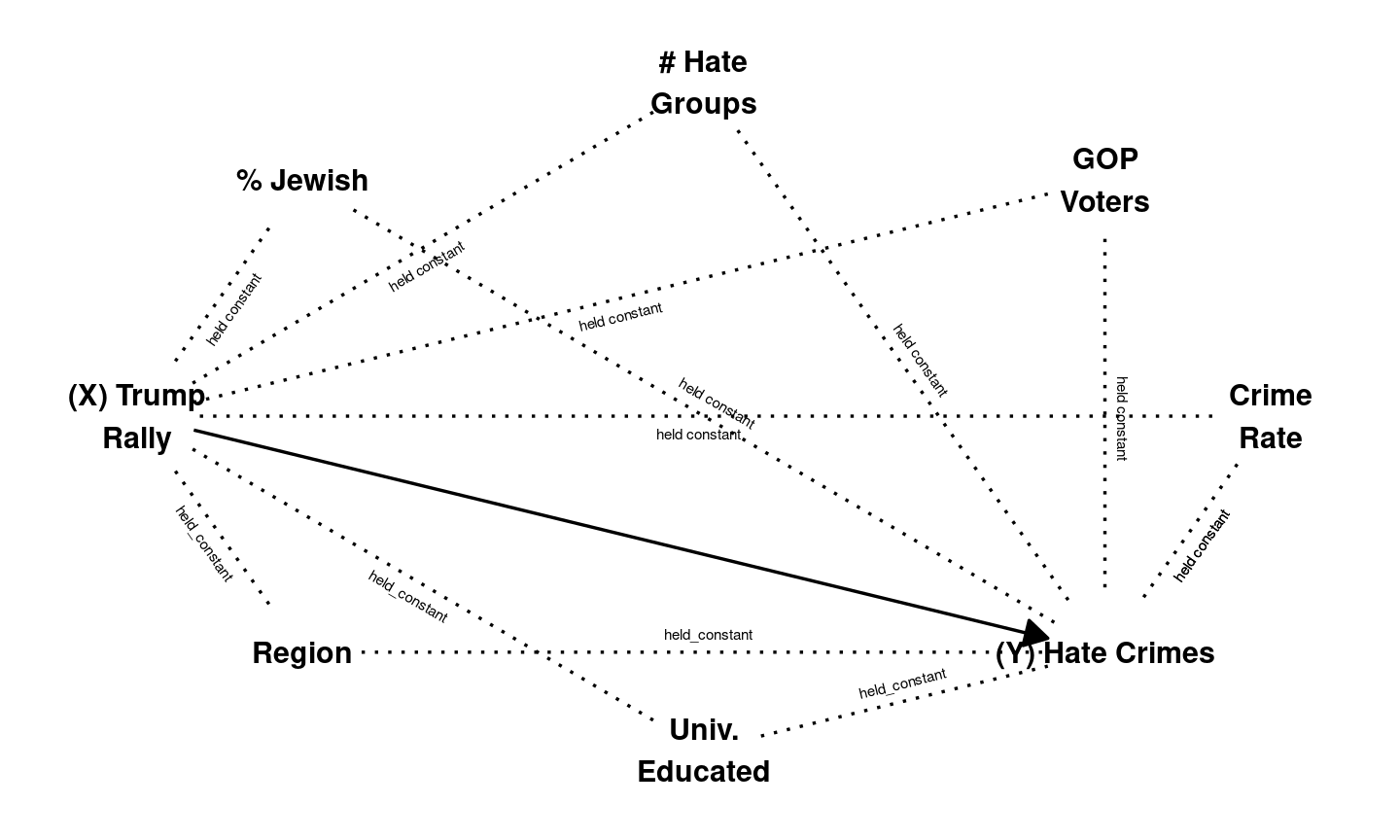April 1, 2021

## Solutions to Confounding

1. Recap
2. Conditioning

## Solutions to Confounding## Solutions to Confounding

Every way of using correlation as evidence for causality makes assumptions

• FPCI cannot be solved without assumptions
• With assumptions, can say confounding/bias is not a problem

## Internal vs External Validity

Every way of using correlation as evidence for causality makes trade-off between:

• internal validity: how plausible are assumptions that set aside confounding?
• external validity: how much does this causal relationship speak to the causal claim/question of interest?
Solution How Bias
Solved
Which Bias
Removed
Assumes Internal
Validity
External
Validity
Experiment Randomization
Breaks $$W \rightarrow X$$ link
All confounding variables $$X$$ is random
Change only $$X$$
High Low
Conditioning Hold confounders
constant
? ? Low High

## Conditioning

### conditioning

when we observe $$X$$ and $$Y$$ for multiple cases, we examine the correlation of $$X$$ and $$Y$$ within groups of cases that are the same on confounding variables $$W, etc. \ldots$$

How does conditioning solve confounding?

• Cases compared have same values on confounding variable $$W$$
• In these groups, $$W$$ cannot affect $$X$$ or $$Y$$
• “Backdoor” path from $$X$$ to $$Y$$ is “blocked”

## Conditioning: Example

A few weeks back we asked:

### Did Trump rallies increase hate crimes?

• inflammatory rhetoric $$\xrightarrow{?}$$ violence
• many argue there is a link
• but is there empirical evidence of causality?

## Conditioning: Example## Conditioning: Example

Correlation between Trump Rallies and Hate Crimes might suffer from confounding

• Places that Trump visits might be different than places he does not.
• These differences might be related to Hate Crimes.## Example: Conditioning:

Feinberg, Branton, and Martinez-Ebers compare hate crimes in counties with and without Trump rallies, but condition on (hold constant):

• percent Jewish
• number of hate groups
• crime rate
• 2012 Republican vote share
• percent university educated
• region